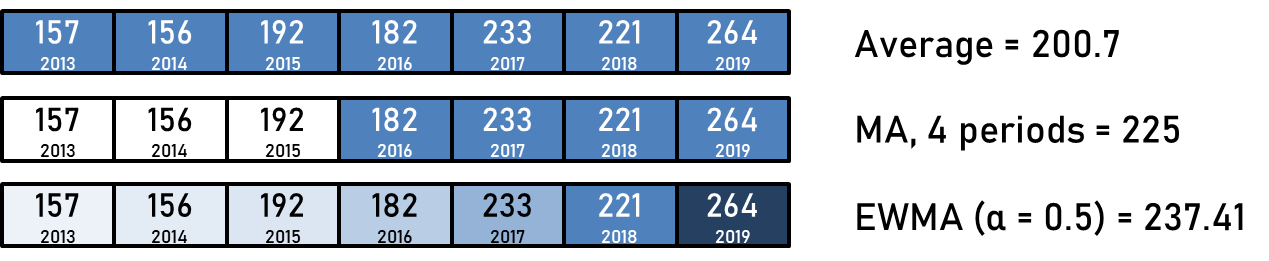# Detecting Progress in SequencesI often try to evaluate whether something that is difficult to measure directly and clearly has improved. For example, I might like to evaluate how my German or logic puzzle skills have changed over time. I could try German past exams or look at logic contest results – however, one problem with these is that there is a lot of noise. For example, for the logic contest results, a score could be artificially low because I had a bad day or the contest was unexpectedly hard; it could also be high because I made multiple lucky guesses on difficult puzzles. Thus, a single measurement is unlikely to be sufficiently reliable.

One solution is then to use one’s average score, or take other statistical summaries of multiple data points. However, we probably don’t want to consider all of the data points we have as equally important. For example, I ranked 155th in a Sudoku GP contest in late 2017 – if I’m trying to evaluate my skill level at the end of 2019, that’s probably not relevant.

We could pick a cut-off point (for example, the beginning of 2019, or the last ten contests) and then discard all of the data from before that, and then simply treat the remaining data as equally important. This is often the basis of sliding window algorithms; if we say that we’re interested in one’s average score from the last ten contests, we can find this metric over time by considering a part of the list ending at today. There are methods for calculating these metrics efficiently (taking time linear in the length of the data stream).

Unfortunately, choosing a suitable window can be difficult – small windows can be vulnerable to noise, while large ones may fail to account for trends present within an individual window. As far as I know, this selection is more of an art than a science.

We can use more complicated approaches as well. Instead of picking a hard cut-off, where data from before the cut-off is irrelevant, we can instead treat data points as becoming less relevant over time. A method that’s often used is exponential weighting; giving the most recent observation a weight of$0 < \alpha < 1$, the second most recent a weight of$\alpha (1 - \alpha)$, the third$\alpha (1 - \alpha)^2$ and so on. As$\alpha$ approaches 0, we approach a simple historical average; as$\alpha$ approaches 1, we approach remembering just the most recent element. I’m not sure if the underlying assumption that events become exponentially less relevant over time is appropriate.

In spite of possibly sounding complex, this method does have computationally favourable properties. If we’re keeping track of a stream of data, we don’t actually need more than constant additional memory. It’s enough to keep just the previous reported average, because incorporating a fresh data point$D$ into our metric can be done by$S_{new} = \alpha D + (1 - \alpha) S_{old}$.

There are some dangers here as well. The first challenge is bootstrapping; how should one pick the initial value of$S$? One could use the first observation, or perhaps an average of the first few observations if short-term divergence from reality is unacceptable.

I think there’s also a risk with massive outliers massively skewing the average (e.g. an API call which usually takes nanoseconds exceptionally taking an hour because of a system outage). This exists with any statistical technique, but if$\alpha$ is small, our estimate will be “corrupted” by the exceptional data even after quite a few additional measurements. With the sliding window method, once the window has expired, the exceptional data point drops out.

In general, the methods we’ve covered assign weighting functions to the data points – the simple average just assigns the same weight to everything, the sliding window assigns the same weight to everything in the window and 0 to things outside the window, while the exponentially weighted moving average (EWMA) weights each point differently based on how recent it is.As an extension, there are techniques for maintaining constant-size reservoirs of values that can be used to approximate more general summaries like order statistics, standard deviations or skewness. These often rely on holding a subset of the values being observed in memory. The selection mechanism for which values should be kept can be written to bias towards more recent measurements. In some ways, the calculation of our standard sliding-window based moving average can be implemented as a special case of this, where new entries are always included, and the oldest entry at each insertion is evicted. That said, we would probably not do this for just an average, as we can do that with constant memory (just remember the current average).

It’s not a particularly scientific or deterministic method, but in practice I find it useful to consider graphs with various transforms on top of them and draw conclusions based on that. I don’t have the sufficient statistical background or intuition to decide beforehand what would work well, unfortunately.